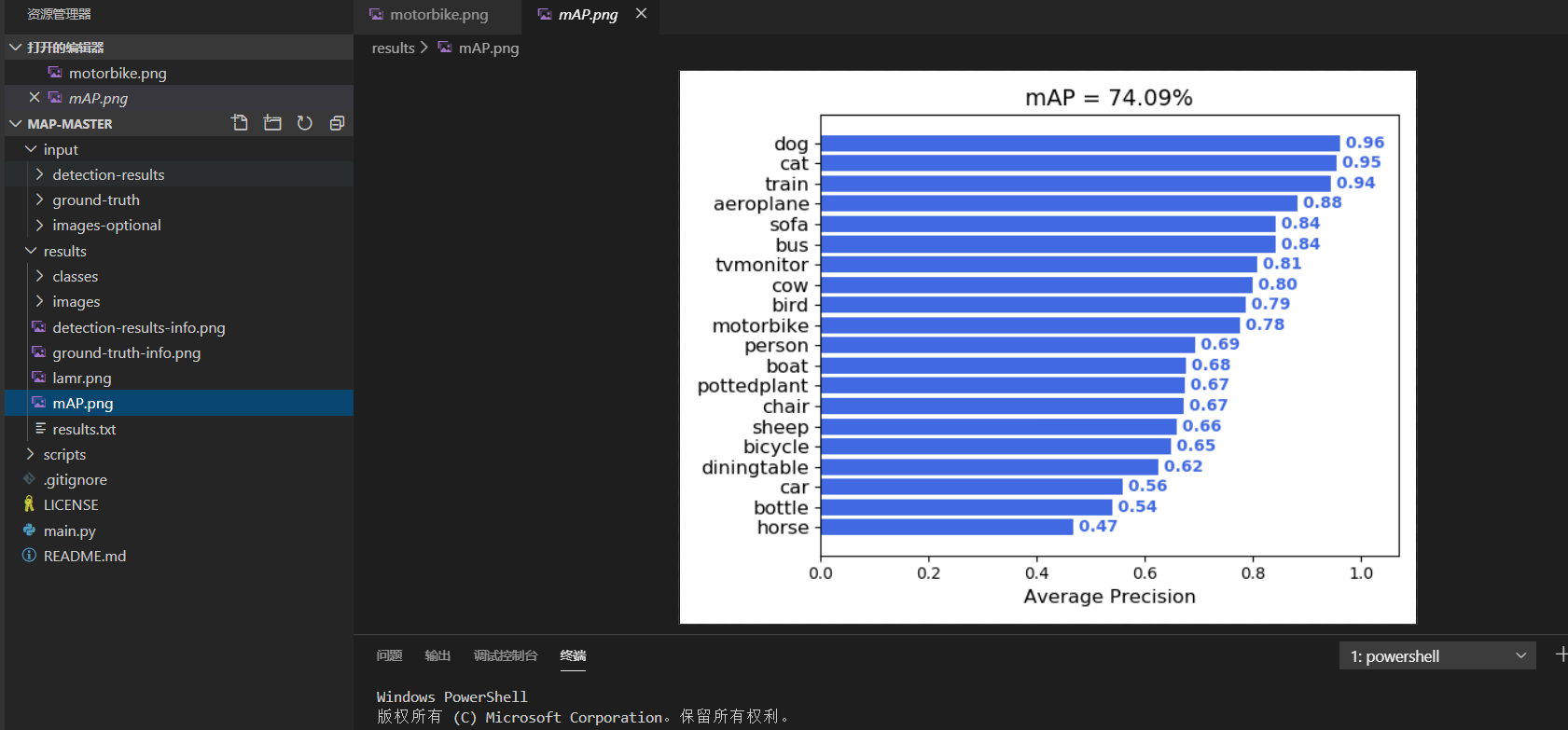# 学习前言# GITHUB代码下载

https://github.com/Cartucho/mAP

https://github.com/bubbliiiing/count-mAP-txt

# 知识储备

## 1、IOU的概念

IOU的概念应该比较简单，就是衡量预测框和真实框的重合程度。## 2、TP TN FP FN的概念

TP TN FP FN里面一共出现了4个字母，分别是T F P N。
T是True；
F是False；
P是Positive；
N是Negative。

T或者F代表的是该样本 是否被正确分类。
P或者N代表的是该样本 被预测成了正样本还是负样本。

TP（True Positives）意思就是被分为了正样本，而且分对了。
TN（True Negatives）意思就是被分为了负样本，而且分对了，
FP（False Positives）意思就是被分为了正样本，但是分错了（事实上这个样本是负样本）。
FN（False Negatives）意思就是被分为了负样本，但是分错了（事实上这个样本是这样本）。

## 3、precision（精确度）和recall（召回率）

P r e c i s i o n = T P T P + F P Precision= \frac{TP}{TP+FP}
TP是分类器认为是正样本而且确实是正样本的例子，FP是分类器认为是正样本但实际上不是正样本的例子，Precision翻译成中文就是“分类器认为是正类并且确实是正类的部分占所有分类器认为是正类的比例”。

R e c a l l = T P T P + F N Recall= \frac{TP}{TP+FN}
TP是分类器认为是正样本而且确实是正样本的例子，FN是分类器认为是负样本但实际上不是负样本的例子，Recall翻译成中文就是“分类器认为是正类并且确实是正类的部分占所有确实是正类的比例”。

## 4、概念举例P r e c i s i o n = 2 2 + 2 = 1 / 2 Precision= \frac{2}{2+2} = 1/2
R e c a l l = 2 2 + 1 = 2 / 3 Recall= \frac{2}{2+1} = 2/3

## 5、单个指标的局限性P r e c i s i o n = 1 1 + 0 = 1 Precision= \frac{1}{1+0} = 1
R e c a l l = 1 1 + 2 = 1 / 3 Recall= \frac{1}{1+2} = 1/3

P r e c i s i o n = 3 3 + 3 = 1 / 2 Precision= \frac{3}{3+3} = 1/2
R e c a l l = 3 3 + 0 = 1 Recall= \frac{3}{3+0} = 1

# 什么是AP

AP事实上指的是，利用不同的Precision和Recall的点的组合，画出来的曲线下面的面积。mAP就是所有的类的AP值求平均。

# 绘制mAP

https://github.com/Cartucho/mAP

detection-results：指的是预测结果的txt。ground-truth：指的是真实框的txt。image-optional：指的是图片，有这个可以可视化，但是这个可以没有。https://github.com/bubbliiiing/count-mAP-txtget_dr_txt.py是用来检测测试集里面的图片的，然后会生成每一个图片的检测结果，我重写了detect_image代码，用于生成预测框的txt。get_dr_txt.py是用来获取测试集中的xml，然后根据每个xml的结果生成真实框的txt。